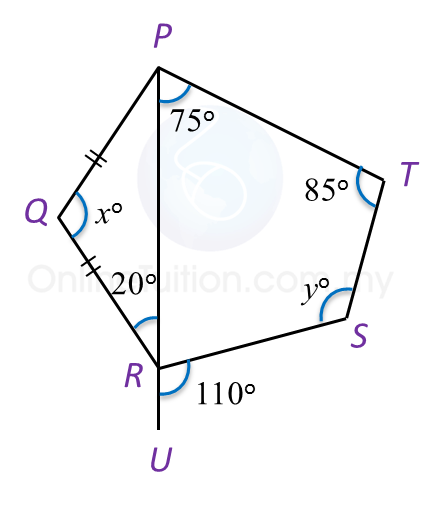# 4.2.2 Polygons, PT3 Practice

Question 4:
In the diagram below, KLMNP is a regular pentagon. LKS and MNQ are straight lines.Find the value of x + y.

Solution:
$\begin{array}{l}\text{Size of each interior angle of a regular pentagon}\\ =\frac{\left(5-2\right)×{180}^{o}}{5}\\ ={108}^{o}\\ \angle PKS=\angle PNQ={180}^{o}-{108}^{o}={72}^{o}\\ \text{Reflex angle}\angle KPN={360}^{o}-{108}^{o}={252}^{o}\end{array}$

$\begin{array}{l}\text{Sum of interior angles of a hexagon}\\ =\left(6-2\right)×{180}^{o}\\ ={720}^{o}\\ \therefore {x}^{o}+{y}^{o}+{72}^{o}+{252}^{o}+{72}^{o}+{100}^{o}={720}^{o}\\ {x}^{o}+{y}^{o}={720}^{o}-{496}^{o}\\ \text{}={224}^{o}\\ \text{}x+y=224\end{array}$

Question 5:
In Diagram below, PQR is an isosceles triangle and PRU is a straight line.Find the value of x + y.

Solution:
$\begin{array}{l}{x}^{o}={180}^{o}-{20}^{o}-{20}^{o}={140}^{o}\\ \angle PRS={180}^{o}-{110}^{o}={70}^{o}\\ {y}^{o}+{85}^{o}+{75}^{o}+{70}^{o}={360}^{o}\\ {y}^{o}+{230}^{o}={360}^{o}\\ \text{}{y}^{o}={130}^{o}\\ {x}^{o}+{y}^{o}={140}^{o}+{130}^{o}\\ \text{}={270}^{o}\\ \text{}x+y=270\end{array}$

Question 6:
In diagram below, PQRSTU is a regular hexagon QUV is a straight line.Find the value of x.

Solution: